# 1- Various sequence to sequence architectures

## 1.1- Basic Models

seq2seq模型主要来源于如下两篇论文：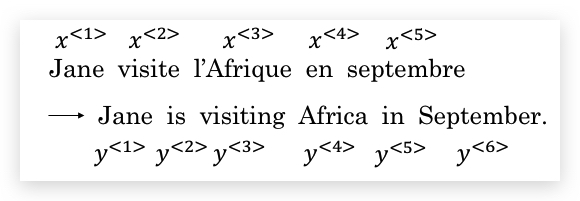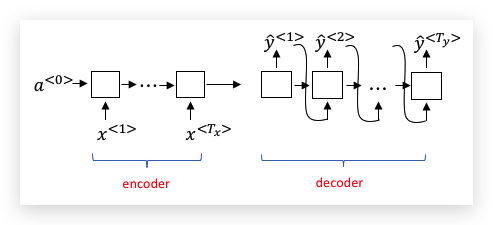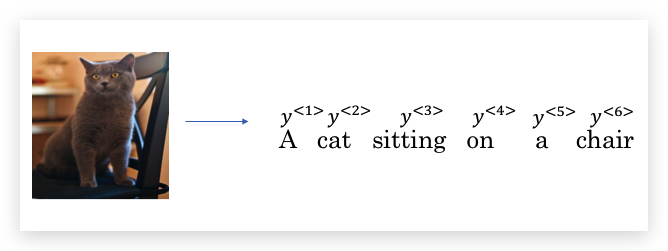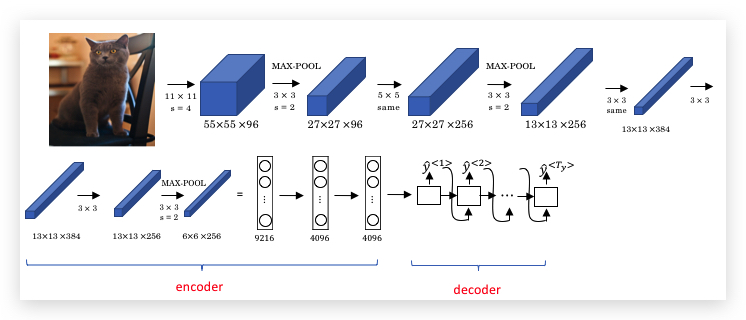## 1.2- Picking the most likely sentence

seq2seq翻译模型和先前讨论的语言模型既有些相似，也有很大不同。我们可以将机器翻译看做构建一个条件语言模型（conditional language model）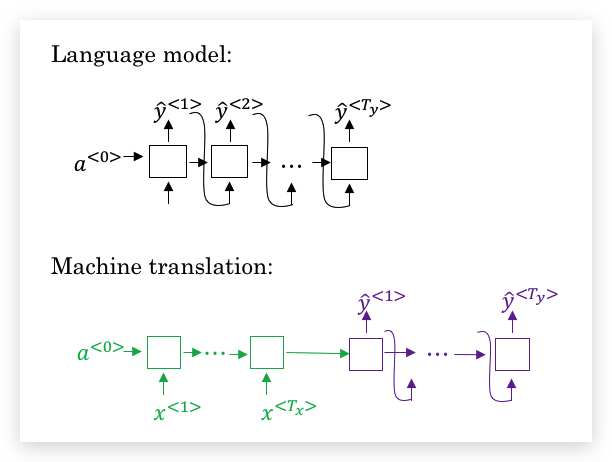• 首先看第一个单词的条件概率$$P(\hat y^{<1>}|x)$$，并选择概率最大的3个单词作为候选，这里个数3称为集束宽（beam width），代表解码器中每个时间步候选的单词个数，记作$$B$$。具体上面的例子，可能选到了3个候选单词是：in, jane, september。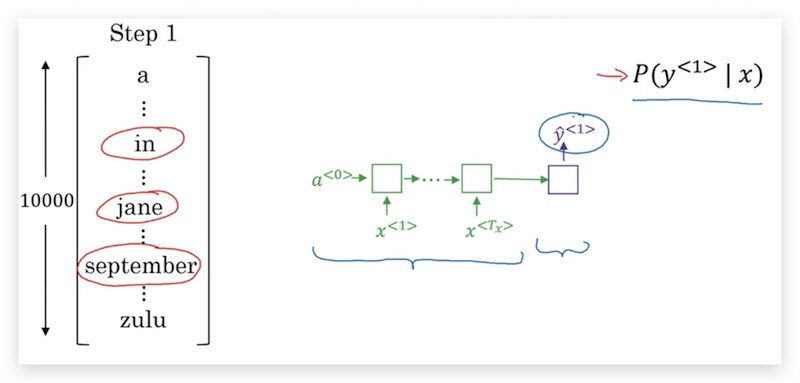• 然后分别考虑第1个单词是上面3个候选单词之一的条件下，第2个单词的概率$$P(\hat y^{<2>}|x, \hat y^{<1>})$$。比如第一个单词是in的概率$$P(\hat y^{<2>}|x, \text{‘in’})$$。然后再选出构成前两个单词概率最大的3个组合，其概率计算为： $% }, \hat y^{\<2>}|x) = P(\hat y^{\<1>}|x) P(\hat y^{\<2>}|x, \hat y^{\<1>}) %]]>$ 由于$$B=3$$，因此我们在这一步会考虑3x10000种组合（第一步对应3个单词，第二步对应词汇表10000个单词），并选择其中概率最大的3个。最终可能的候选是：in september, jane is, jane visits（第一个单词是september的情况已经被剔除了）。我们将30000的搜索范围，收缩为3个候选。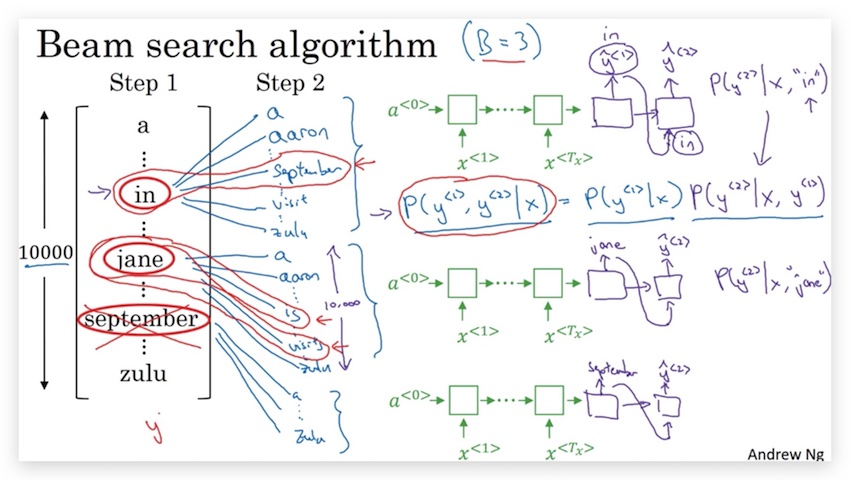• 第3步和第2步类似，可能继续得到3个候选：in september jane, jane is visiting, jane visits africa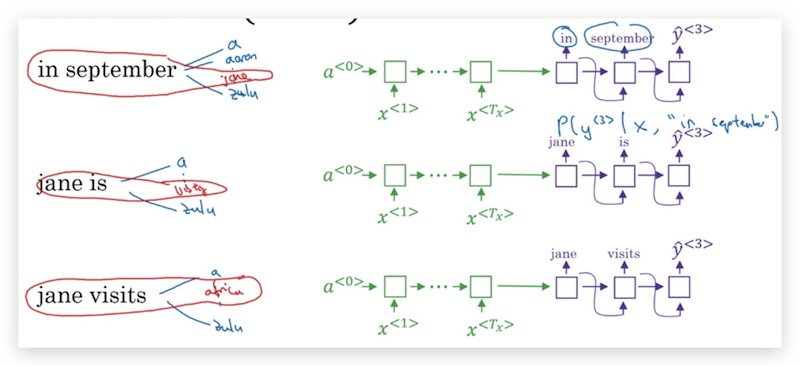1. 长度归一化（length normalization）

1. 如何选择参数$$B$$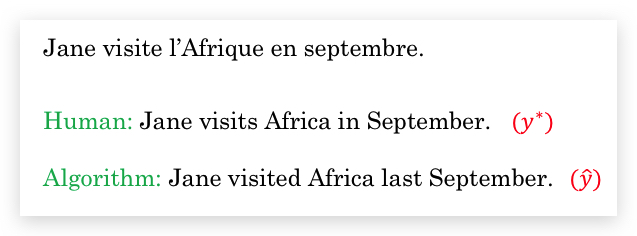方法很简单，直接用RNN去计算人类翻译和算法翻译的概率，即比较$$P(y^* x)$$和$$P(\hat y x)$$做判断： * 如果$$P(y^* x) > P(\hat y x)$$，说明beam search效果不好，没有搜索到概率更大的翻译语句。 * 如果$$P(y^* x) \le P(\hat y x)$$，说明RNN效果不好，较好的翻译语句没有得到更大的概率。

## 1.6- Bleu Score

TODO: 衡量机器翻译结果和人工翻译结果的相似性（重合性） 如果只翻译出一个单词呢？ 这一集的视频太水了，都懒得剪辑了。

## 1.7- Attention Model Intuition

1. 长序列问题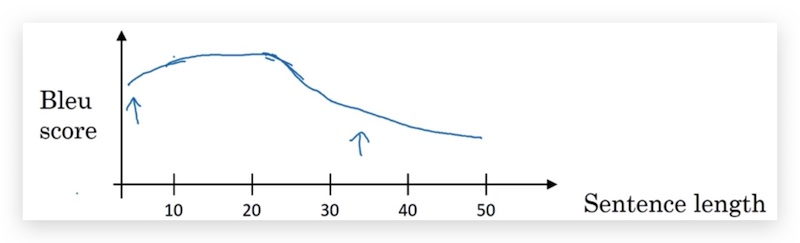1. Attention model

（我有个疑问，为什么一定要和人一样，人是因为有记忆限制的缺陷。如果不用注意力模型，翻译效果会不会比人更好？）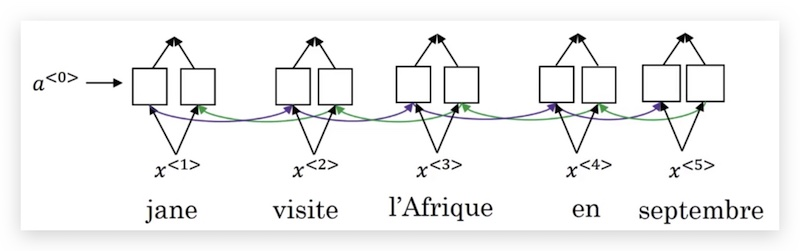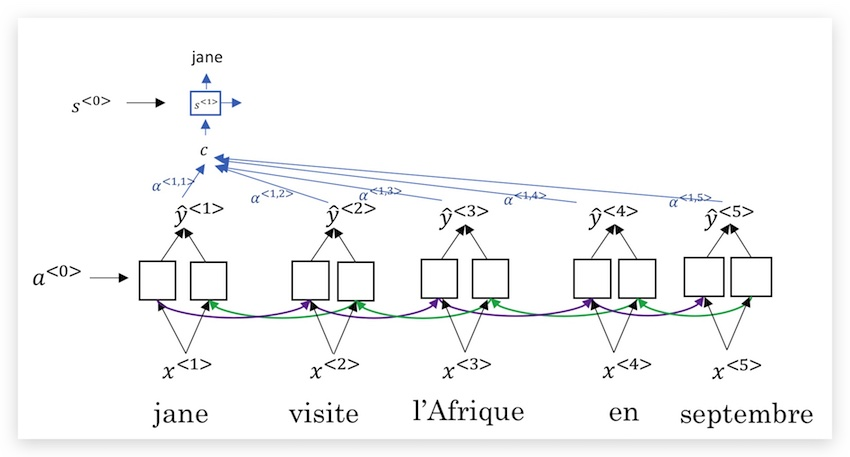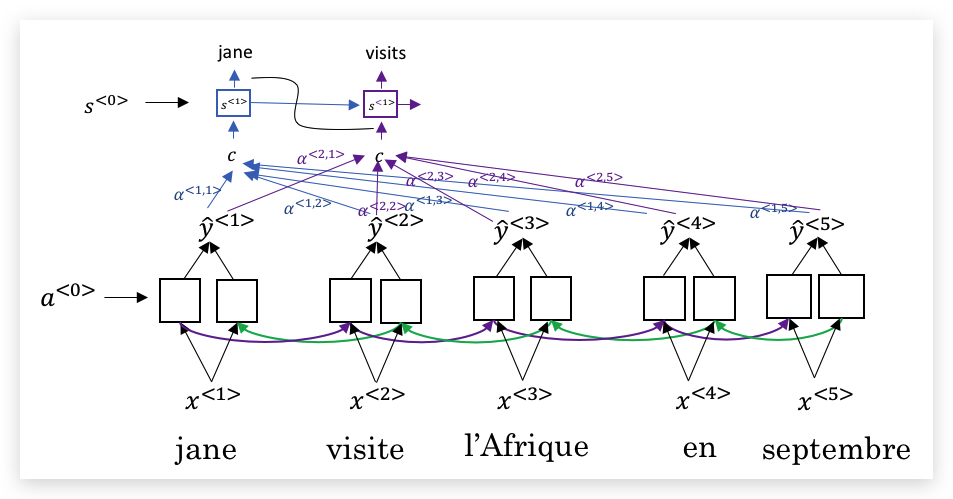TODO: 注意力模型的思想主要来源论文：[Bahdanau et. al., 2014. Neural machine translation by jointly learning to align and translate]

## 1.8- Attention Model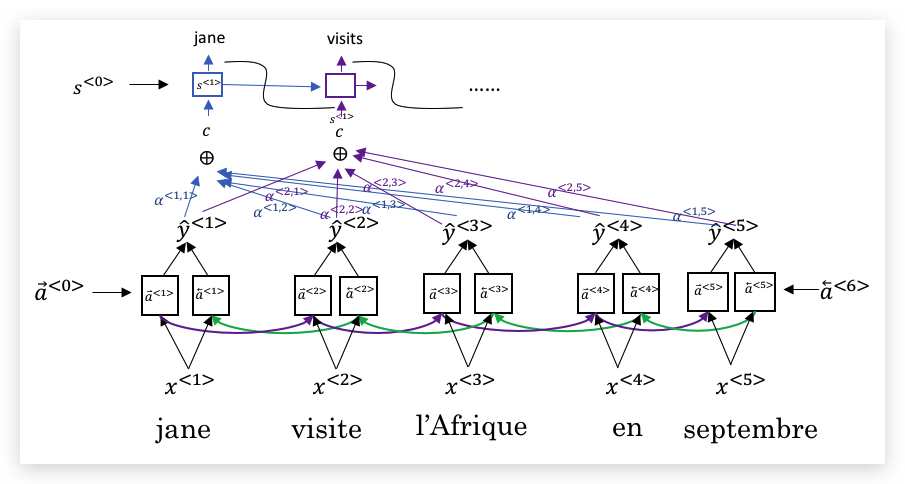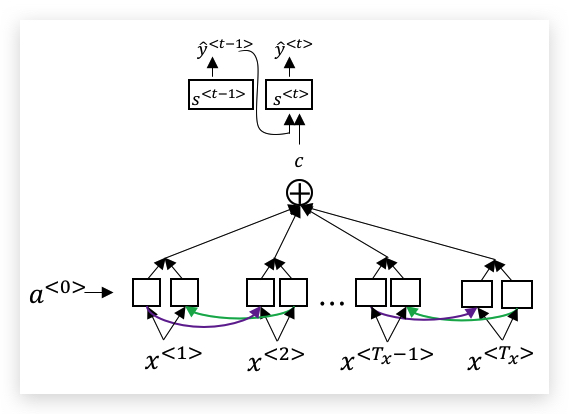$$\alpha^{<t,t’>}$$，即代表了$$y^{<t>}$$对$$a^{<t’>}$$的注意力大小。这个权重的计算如下：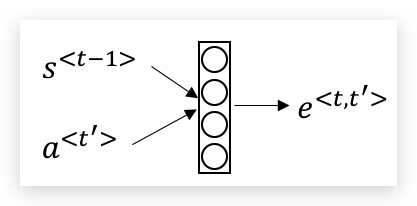# 2- Speech recognition - Audio data

## 2.1- Speech recognition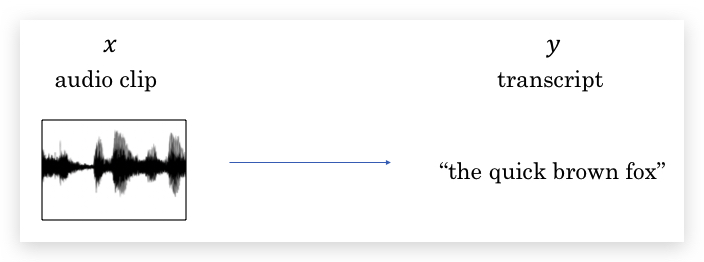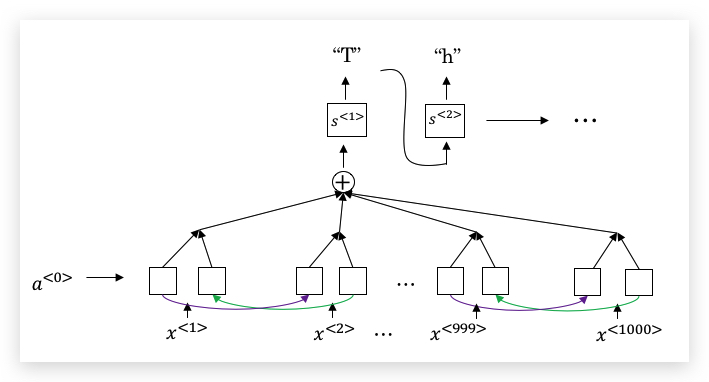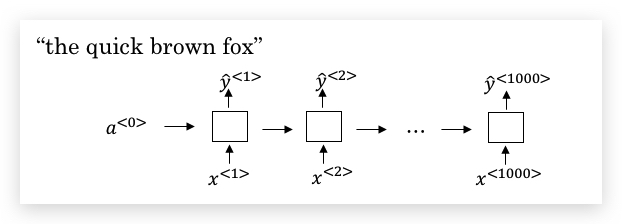ttt_h_eeee_ _ _ <space> _ _ _qqq_ _ _……

the quick brown fox

## 2.2- Trigger Word Detection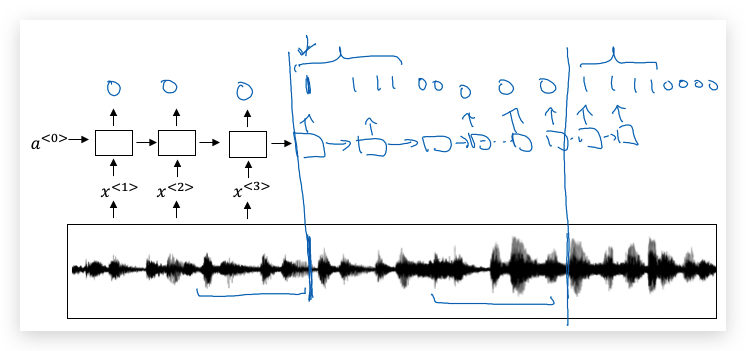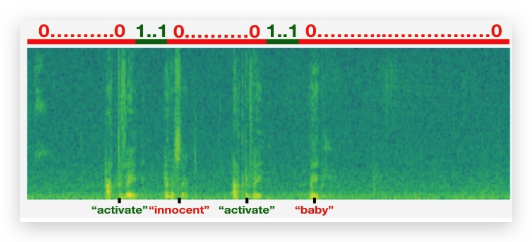# 3- Conclusion

## 3.1- Conclusion and thank you

deeplearning.ai课程结束了！与开篇的AI is the new electricity的口号相呼应，Andrew Ng激励我们：

Deep learning is a super power! If that isn’t a superpower I don’t know what is.-------------------------